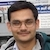# NAEST 2019 Screening Test: Surface TensionBy

The surface tension is responsible for many interesting phenomenon. NAEST questions given here are related to the surface properties of the fluids.

## Question from NAEST 2019

Question: Talcum powder was displaced because,

1. The density of the powder is smaller than the density of the soap solution.
2. The density of the soap solution is smaller than the density of the powder.
3. The surface tension of the soap solution is smaller than that of water.
4. The evaporation of the soap solution is faster than that of water.

Answer: The surface tension of the soap solution is smaller than that of water.

## Question from NAEST 2017

Question 1: The cap floats in water. In the first part, the cap comes to the edge,

1. Because of the attraction from the rim
2. Because of the force of buoyancy
3. Because of the surface tension
4. Because of its weight

Answer: Because of the surface tension

Question 2: In the second part, the cap comes at the middle of the water

1. Because of the repulsion from the rim
2. Because of the force of buoyancy
3. Because of the surface tension
4. Because of its weight

Answer: Because of the surface tension

## Question from NAEST 2014

Question: Why a drop of water makes the slides stick together?

Answer: The adhesive force between glass-water and cohesive force between water-water molecules is responsible for sticking of slides. This can be explained through the phenomenon of surface tension (pressure difference created by the surface tension).